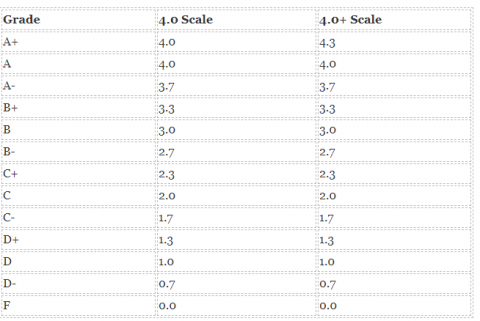### How to Calculate GPA Using Different MethodsShare

Grade point average (GPA) is a score average calculated on the letter grades you make each semester. Each letter grade is given a numerical value from 0-4 or 5 points; it varies subject to your institution’s scale. Schools also look at cumulative GPAs, which indicate your average GPA over past few years of schooling, when you graduate or apply to an institute of higher studies.
It is most likely, that in your high school classes, your final finishing grades are identified as alphabetical letters (A-, B+, etc.) or as percent (92, 85, etc. out of 100). A GPA, first translates those letters or percent into numbers, and then averages these numbers collectively.

GPA is practiced across the US, Canada and Australia. However, the grading scale is not the same for every country. In the US and Canada, the GPA band is 0.0 to 4.0 points, with 4 being the highest mark/score, although few schools in Australia use a GPA band from 0.0 to 7.0, with 7 being the highest mark/score.

Different Methods of Calculating GPA
Higher schools in the U.S. use different methods of computing point average because sometimes these systems are a little different from each other. Both 4.0 Scale and 4.0+ Scale are used. Confirm which scale is implemented in college you enter. Now let’s study how GPA is calculated by exercising different methods.

Simple Method

Now let’s compute grade point average using 4.0+ Scale system. In contrast to 4.0 scale, 4.0+  system varies by credits allocated

 Grade 4.0 Scale 4.0+ Scale A+ 4.0 4.3 A 4.0 4.0 A- 3.7 3.7 B+ 3.3 3.3 B 3.0 3.0 B- 2.7 2.7 C+ 2.3 2.3 C 2.0 2.0 C- 1.7 1.7 D+ 1.3 1.3 D 1.0 1.0 D- 0.7 0.7 F 0.0 0.0

As you can comprehend from the above table, determining grade point average using 4.0+ Scale system will be different if a student has excellent accomplishments.

Weighted Point Average
Presently, many schools measure grade point average with the help of 4.0+ scale as they use weighted scale. Weighted value is calculated taking into account quite a few factors like assignments difficulty, level or class. For example, students from elementary school having all “A” grades will obtain 4.0, while students from high school getting all “A” marks will achieve 5.0. The classifications of challenging courses are also different in each school. If school offers AP or IB classes, this means that Honors classes are available. Extra difficult classes have additional credits. As a result, 4-credit class is valued more than 2-credit class. Your schedule may look like this:

 Class Credits Grades Math 2 A(4.0) History 2 B+(3.3) Chemistry 4 A(4.0) Physical Education 1 C-(1.7) German 2 B(3.0) Literature 1 B-(2.7) Physics 1 A(4.0) TOTAL 13

By looking at the above table, you may observe the following:

• You’re excellent at exact sciences
• Physical education is not your strong point
• You earned 13 total credit points in total during the particular academic year

• Multiply numeric grades by credits one by one
• Add the numbers you’ve got together
• Divide your total score by total credits i.e. (45/13)
• Get your score that equals 3.46

 Grade With AP Without AP A+ 5.3 4.3 A 5.0 4.0 A- 4.7 3.7 B+ 4.3 3.3 B 4.0 3.0 B- 3.7 2.7 C+ 3.3 2.3 C 3.0 2.0 C- 2.7 1.7 D+ 2.3 1.3 D 2.0 1.0 D- 1.7 0.7 F 0.0 0.0

If you want to calculate weighted value with AP, use the same formula. You only should work out weighting AP classes, but not credits. If you apply the same data to calculate for Honor Classes, make use of this method:

5 + 3.67 + 4.67 + 4 + 3 = 20.34

GPA = score/classes = 20.34/5 = 4.06

Unweighted Point Average:

When calculating unweighted value, students don’t have to consider their classes and levels. If, for example, one has “A” in two subjects and “B” on three subjects, “A” is translated to “4.0,” and “B” is translated to “3.0”:

Unweighted GPA = (Point for course 1 + Point for course 2 +…)/Number of classes

Unweighted GPA = (4.0 + 4.0 + 3.0 + 3.0 + 3.0)/5 = 3.4

Some colleges use more specific grading systems when they assess students on a 0-100 scale instead of using letters. In this event, a student who’s got 90 points on average would have point average of 3.5. However, another student having 92 points would get the value of 3.7. This gives a chance to evaluate caliber of students in a just a few easy steps.

How to calculate unweighted GPA

The first and foremost thing to do in order to evaluate a grade point average is to convert each of the final class grades you’ve achieved until now in high school into the right decimal.

Here is the standard unweighted scale for achieving this:

 Letter Grade Percentile GPA A+ 97-100 4.0 A 93-96 4.0 A- 90-92 3.7 B+ 87-89 3.3 B 83-86 3.0 B- 80-82 2.7 C+ 77-79 2.3 C 73-76 2.0 C- 70-72 1.7 D+ 67-69 1.3 D 65-66 1.0 F Below 65 0.0

Then, execute the calculation in the following sequence: